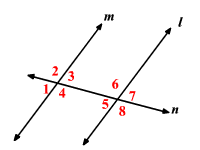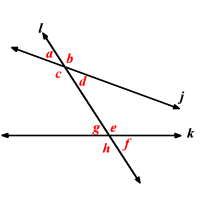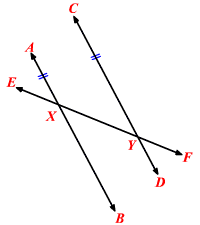# Transversal

In geometry, a  transversal  is a line that intersects two or more other (often  parallel ) lines.

In the figure below, line  $n$  is a transversal cutting lines  $l$  and  $m$ .When two or more lines are cut by a transversal, the angles which occupy the same relative position are called corresponding angles .

In the figure the pairs of corresponding angles are:

When the lines are parallel, the corresponding angles are congruent .

When two lines are cut by a transversal, the pairs of angles on one side of the transversal and inside the two lines are called the consecutive interior angles .

In the above figure, the consecutive interior angles are:

If two parallel lines are cut by a transversal, then the pairs of consecutive interior angles formed are supplementary .

When two lines are cut by a transversal, the pairs of angles on either side of the transversal and inside the two lines are called the alternate interior angles .

In the above figure, the alternate interior angles are:

If two parallel lines are cut by a transversal, then the alternate interior angles formed are congruent .

When two lines are cut by a transversal, the pairs of angles on either side of the transversal and outside the two lines are called the alternate exterior angles .

In the above figure, the alternate exterior angles are:

If two parallel lines are cut by a transversal, then the alternate exterior angles formed are congruent .

Example 1:In the above diagram, the lines $j$ and $k$ are cut by the transversal $l$ . The angles $\angle c$ and $\angle e$ are…

A. Corresponding Angles

B. Consecutive Interior Angles

C. Alternate Interior Angles

D. Alternate Exterior Angles

The angles $\angle c$ and $\angle e$ lie on either side of the transversal $l$ and inside the two lines $j$ and $k$ .

Therefore, they are alternate interior angles.

The correct choice is $C$ .

Example 2:In the above figure if lines $\stackrel{↔}{AB}$  and $\stackrel{↔}{CD}$ are parallel and $m\angle AXF=140°$  then what is the measure of $\angle CYE$ ?

The angles $\angle AXF$  and $\angle CYE$  lie on one side of the transversal $\stackrel{↔}{EF}$ and inside the two lines $\stackrel{↔}{AB}$ and $\stackrel{↔}{CD}$ . So, they are consecutive interior angles.

Since the lines $\stackrel{↔}{AB}$ and $\stackrel{↔}{CD}$  are parallel, by the consecutive interior angles theorem $\angle AXF$  and $\angle CYE$  are supplementary.

That is, $m\angle AXF+m\angle CYE=180°$ .

But, $m\angle AXF=140°$ .

Substitute and solve.

$\begin{array}{l}140°+m\angle CYE=180°\\ 140°+m\angle CYE-140°=180°-140°\\ m\angle CYE=40°\end{array}$